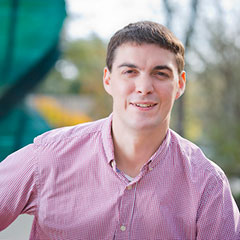Select Page# School of Science & Engineering

### Mathematics

Students in mathematics at Anderson University are taught how to solve problems and see how mathematics changes the way that we look at the world. As a student progresses through the major, students learn how to do complex calculations and the theorems behind these calculations.

Apply Now

### AU offers two degrees in mathematics.

• BA in Mathematics
• BS in Mathematics
• Both can be completed in as little as 3 years.

### What courses will I take?

• Calculus I, II, and III
• Linear Algebra
• Problem Seminar

For the B.A. degree, there will be 30 hours of coursework with additional courses in mathematics. These are chosen to complement the student’s mathematics interests and objectives through consultation with the student’s department advisor. View the courses required for the Mathematics, B.A. For the B.S. degree, there will be 47 hours of coursework that includes courses in physics, chemistry, and programming and prepares students for graduate-level study. View the courses required for the Mathematics, B.S.

### What kind of jobs can I anticipate after graduation?

• biomathematics
• education
• operations research
• actuarial science
• finance
• mathematical modeling
• statistics
##### Mathematics Student Research

The Anderson University Department of Mathematics offers a unique, hands-on research experience.   Undergraduate students work alongside faculty to conduct original research in mathematics.  This exciting research into new mathematics is presented in a variety of venues.  These have included poster sessions and invited talks at other universities.  Opportunities such as these advance our knowledge about God’s creation and develop skills that are essential for students furthering their studies at the graduate level.

Here are samples of the projects that are currently underway:

Adventures in the Quantum Polynomial Ring:  Linear Algebra Computations in C Abstract:

• The p-polynomials appear as the elements of transition matrices used to convert a special class of bases to the standard basis within the quantum polynomial ring. We examined computational methods for generating p-polynomials. An algorithm for finding a p-polynomial has been known; however, the implementation of this algorithm was not sufficiently fast. Through algorithmic analysis, a change in the implementation language, and the adoption of matrix-based algorithms, significant improvements in speed were realized. Optimization of this process has led to a speedup of over 140,000 times.
• Linear Algebra Computations in C Research Project [PDF]

Adventures in the Quantum Polynomial Ring: Patterns in the p-Polynomials Abstract:

• The p-polynomials appear as the elements of transition matrices used to convert a special class of bases to the standard basis within the quantum polynomial ring. The purpose of this study is to analyze these polynomials for patterns and eventually catalog these newly generated p-polynomials for future analysis. The initial strategy for finding these patterns will take advantage of generalized rules from the modified R-polynomials and Kazhdan-Lusztig polynomials. Additional strategies arise by observing new patterns from the list of computer-generated polynomials. We also examine a special class of p-polynomials that are generated by the longest word in the symmetric group and describe patterns in the coefficients of these polynomials.
• Patterns in the p-Polynomials [PDF]

On the Creation of Rank Two Centrosymmetric Matrices

• For any square matrix B, we can create a centrosymmetric matrix A = B+JBJ where J is the skew identity matrix. If the matrix B is created as the outer product of two vectors v and h, the resulting centrosymmetric matrix has a maximal rank of 2. However, not all such rank two matrices can be written in this form. In this work, we fully examine when a 3 x 3 centrosymmetric matrix can be created from two vectors and generalize our results to larger matrices.
• Creation of Rank Two Centrosymmetric Matrices [PDF]

If you have difficulty accessing the information in the above PDF files, please contact us.

##### Mathematics Facilities

Located on the third floor of Decker Hall, the AU Department of Mathematics is an open and inviting space.  Four faculty offices open into a common area with whiteboard walls. All who pass through can see mathematics being done.

Decker 338 is a classroom that has been specially designed for mathematics courses. Distinctive features include floor to ceiling whiteboard walls and easily movable furniture. Tables in the room provide ample space for notebooks, textbooks, and a laptop. The versatile nature of the furniture allows the room to be transformed to accommodate lectures with 35 students, students working in groups, and smaller seminar based courses.

Decker 330 serves as the department's mathematics lab. This room is used for tutoring, seminar space, and a place where students can work on projects together.

#### OUR FACULTY## Dr. Justin Lambright## Dr. Courtney Taylor